A100, A105, A110 Dan Swearingen

Basic Algebra

1. Cross-multiplication

This is done to isolate an unknown on one side of an equation; that is, you are solving for an unknown. Follow these steps (solving for the y, assume m, n, and x are known quantities):

Note: "solving for y" means setting up the equation so it reads: y = ...

 a.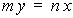our original equation -- we want to solve this for y, b.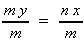Since we know m, n, and x we can now calculate y c.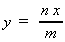divide both sides by m, the m's on the left cancel.

The point is, anything you do to one side you must balance by doing the same thing to the other side.

2. Powers and Roots

Many times in astronomy (e.g., Kepler's third Law), we must raise a number to a power or take its root.

a.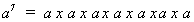b.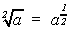the square-root is the same as raising to the 2 power.

c.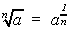the nth root of a.

d.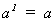e.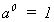any number to the zeroeth power is 1.

f.g.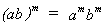e.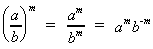f.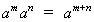g.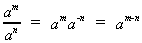h.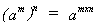i.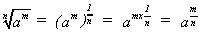Examples: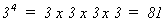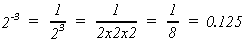3. Scientific Notation (or powers of 10)

Proper scientific notation is: one digit (not equal to zero) followed by a decimal point, followed by any other significant digits, then multiplied times the appropriate power of ten.

Scientific notation is very helpful when doing math in astronomy because we are often dealing with a combination of very large numbers and very small numbers.

 1. = 100 Any number raised to the zeroeth power is 1.0. 10. = 101 0.1 = 10-1 100. = 102 0.01 = 10-2 1,000. = 103 0.001 = 10-3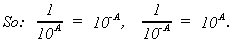The number "one hundred thousand" can be written many ways:

 100,000. = 100000. x 1 Note: as we move the decimal point LEFT one place the power of ten increases ONE. = 100000. x 100 = 10000. x 101 = 1000. x 102 = 100. x 103 = 10. x 104 = 1. x 105 This number is the only on in proper scientific notation.

Similarly:

0.0000007 = 0.000007 x 10-1

= 0.00007 x 10-2

= 0.0007 x 10-3

= 0.007 x 10-4

= 0.07 x 10-5

= 0.7 x 10-6

= 7 x 10-7

Examples:

500. = 5. x 100. = 5 x 102

64,000 = 6.4 x 10,000. = 6.4 x 104

2,356,000. = 2.356 x 1,000,000. = 2.356 x 106

.003 = 3. x .001 = 3 x 10-3

.00045 = 4.5 x .00001 = 4.5 x 10-4 .010906 = 1.0906 x .01 = 1.0906 x 10-2

Multiplication: To multiply powers of 10, add the exponents (the powers).

10A x 10B = 10(A + B)

103 x 102 = 10(3 + 2) = 105

10-4 x 10-6 = 10(-4 - 6) = 10-10

10-16 x 104 = 10(-16 + 4) = 10-12

A x 10a x B x 10b = (A x B) x 10(a + b)

2 x 103 x 3 x 102 = (2 x 3) x 10(3 + 2) = 6 x 105

4.1 x 10-1 x 2.2 x 10-3 = (4.1 x 2.2) x 10(-1 - 3) = 9.02 x 10-4

5 x 10-2 x 4 x 107 = (5 x 4) x 10(-2 + 7) = 20 x 105 = 2 x 101 x 105 = 2 x 106

Division: To divide powers of 10, subtract the exponents.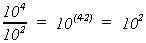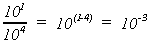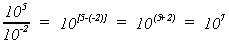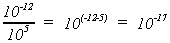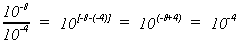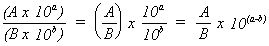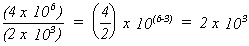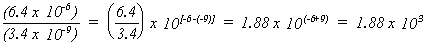4. Significant Figures

Significant figures are any digits in a number which convey information about the number other than placement of the decimal point. Huh? The concept of significant figures is made somewhat more clear by always using scientific notation. Here are some examples:

2,000,000.0 in scientific notation is 2 x 106. Once the number is written in scientific notation it is clear that 2,000,000 has only one significant digit: 2. The zeros do nothing but convey the order of magnitude of the number (the power of ten).

How many significant figures does 2,000,340.0 have?

Six. To see this, write the number in scientific notation: 2.00034 x 106. Only trailing zeros don't count as significant figures.

Pi. If pi is 3.1415927... , how many significant figures does pi have?

An infinite number. In real life we are always using B rounded to some number of significant figures appropriate to the problem we're working on.

In 100-level astronomy classes one or two significant figures are almost always appropriate. Why? Because all too often in astronomy we know a value with poor precision. So keeping all significant figures in calculations is merely fooling yourself into thinking that you have greater precision than you actually do.

So, don't write down every digit your calculations (or your calculator) give you. Round off your results to the same number of significant digits as are found in the number with the least significant digits:

3.0379624 x 10-24 x 2.6 x 10-2 =

= 3.0379624 x 2.6 x 10-24-2

= 7.8987020 x 10-26

(2.6 had only 2 significant digits so anything more than 2 digits in the answer is not real)

= 7.9 x 10-26